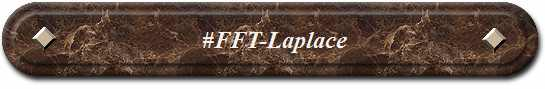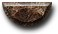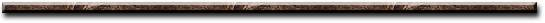News Science Technology Contact Index
 FFT-Laplace What are they? In system engineering, there are two important transforms, Fast Fourier and Laplace. Fast Fourier transform is a tool for signal processing and Laplace is mainly applied to circuit analysis controller design. What do they do? Many of the parameters in our universe interact through differential equations. For example, the voltage across an inductor is proportional to the derivative of the current through the device. Likewise, the force applied to a mass is proportional to the derivative of its velocity. Fast Fourier and Laplace transforms analyze differential equations. How do they work? A system's differential equation is solved by mapping it onto a linear algebraic equation with Laplace Transform. The solution is then mapped back onto the system's differential equation. Fast Fourier transform converts a signal from its original domain (often time and space) to a representation in the frequency domain and vice versa. Applications: Fast Fourier transform is used: to detect seizure problems in EEG electrical signals, to convert 3D images into electrical signals, to convert electrical signals into 3D images for analysis. Laplace transform is used to solve analysis problems in electrical circuits (often filters).[News] [Science] [Technology] [Contact] [Index]
 Contact email: qooljaq@qooljaq.com